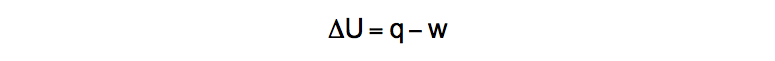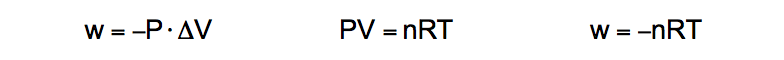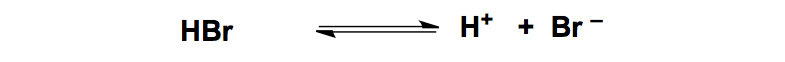Clutch Prep is now a part of Pearson
Ch.6 - Thermochemistry WorksheetSee all chapters

# Enthalpy (IGNORE)

See all sections
Sections
Nature of Energy
Kinetic & Potential Energy
First Law of Thermodynamics
Internal Energy
Endothermic & Exothermic Reactions
Heat Capacity
Constant-Pressure Calorimetry
Constant-Volume Calorimetry
Thermal Equilibrium
Thermochemical Equations
Formation Equations
Enthalpy of Formation
Hess's LawJules Bruno

Enthalpy, ΔH, is an extensive property and state function that represents the total amount of heat within a chemical reaction or system.

Thermochemistry

Internal Energy, work and heat

The First Law of Thermodynamics states that the total energy in an isolated system is constant. The meaning of this definition is that energy can change from one form to another, but never be created nor destroyed. This relationship is illustrated by the equation:Internal Energy of a System

U, sometimes shown as E, represents the internal energy of the system or chemical reaction. The variables of q and w represent heat and work respectively.

Heat and Enthalpy of Reaction

At constant temperature heat, q, is equal to the enthalpy of reaction, ΔHRxnHeat and Enthalpy

Work

Work is the amount of energy required to move an object against an external force. The most common form is pressure-volume work and is represented as:Work Equation

The symbols of P and V represent pressure and volume respectively and by using the Ideal Gas Law we can incorporate moles, the gas constant R, and temperature.Derived work equation

Under constant volume, ΔV = 0. When placing it into the first equation for work we obtain w = 0.

Exothermic vs. Endothermic Processes

In an exothermic process a system or chemical reaction releases heat from the system to the surroundings. This causes the molecules to slow down as they lose thermal energy and begin to form bonds.Exothermic Reaction (Bond Forming)The releasing of thermal energy by the reactant molecules also produces an energy diagram where the products are lower in energy than the reactants.Energy Diagram (Exothermic Process)

In an endothermic process the system absorbs heat from the surroundings. By absorbing their thermal energy, the molecules speed up enough to break their bonds.Endothermic Reaction (Bond Breaking)

The absorbing of thermal energy by the reactant molecules also produces an energy diagram where the products are higher in energy than the reactants.Energy Diagram (Endothermic Process)

Thermochemical Equations

thermochemical equation is a stoichiometric question that now incorporates the heat or enthalpy of a reaction, ΔHRxn. Whereas a normal stoichiometric reaction deals with a mole to mole ratio, a thermochemical equation deals with a ΔH to mole ratio.Stoichiometric vs. Thermochemical

Let’s take a look at a typical thermochemical equation practice question.

PRACTICE: How much heat (in kJ/mol) must be absorbed to produce 35.4 g NH3?Thermochemical Question

STEP 1: Calculate the molecular mass of NH3Calculating Molar Mass (NH3)STEP 2: Convert the grams of NH3 into moles.Grams to Moles Conversion

STEP 3: Perform a mole to ΔH comparison to find the amount of heat released or absorbed.Thermochemical Equation

Using the balanced chemical equation allows you to determine the amount of heat, which in this case will be in units of kilojoules.Mole to Enthalpy ConversionEnthalpy of Formation

In a formation equation 1 mole of a product is formed from the standard states of its elements. ΔH­is used to represent the enthalpy of formation for a compound or element.

For example, the formation of Ca(OH)2 comes from the standard states of carbon, oxygen and hydrogen combining together.Formation Equation

By using the enthalpies of formation for each compound we can calculate the enthalpy of reaction, ΔHRxn, by doing products minus reactantsJules Bruno